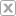# Video Gallery

## Algebra 1 - Slope Intercept Form

Go to Video Gallery Added Apr 22, 2020Copy this URL: Embed code:
Go
Objective 1) Identify Slope and Y-Intercept from an Equation in Slope Intercept Form a) be able to (re)write and equation into Slope Intercept Form, if given a Linear Equation not in Slope Intercept Form 2) Write the Equation of a Line in Slope Intercept Form given a) the slope and y-intercept b) the graph of a line c) two points (that exist) on the line 3) Graph a Line given the Equation of a Line (in Slope Intercept Form)
Show Transcript